## Trace (Matrix)

The trace of anSquare Matrix A is defined by(1)

For Square Matrices A and B, it is true that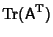(2)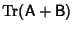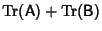(3)(4)

(Lange 1987, p. 40). The trace is invariant under a Similarity Transformation(5)

(Lange 1987, p. 64). Since(6)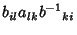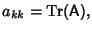(7)

whereis the Kronecker Delta.

The trace of a product of square matrices is independent of the order of the multiplication since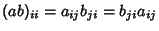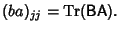(8)

Therefore, the trace of the Commutator ofandis given by(9)

The product of a Symmetric and an Antisymmetric Matrix has zero trace,(10)

The value of the trace can be found using the fact that the matrix can always be transformed to a coordinate system where the z-Axis lies along the axis of rotation. In the new coordinate system, the Matrix is(11)

so the trace is(12)

References

Lang, S. Linear Algebra, 3rd ed. New York: Springer-Verlag, pp. 40 and 64, 1987.